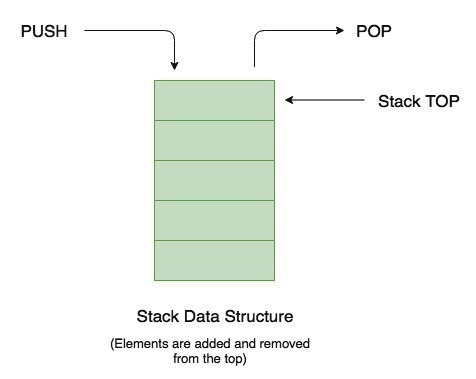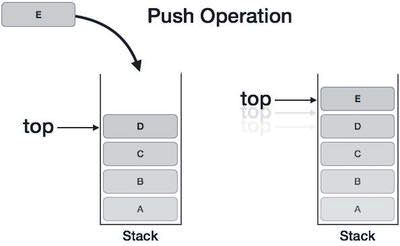×

Search anything:

# Stack in C++ STL

#### C++ Software Engineering standard template library StackFREE BOOK -> Problems for the day before your Coding Interview (on Amazon)

The Stack in the STL of C++ is a dynamically resizing container. Stack class is an container adapter. Containers or container classes store objects and data. There are in total seven standard "first-class" container classes and three container adaptor classes and only seven header files that provide access to these containers or container adaptors. deque container is provided as a default container.

Stack is a data structure that collects data, but has specific operations, which operates in LIFO (Last in First out) context.Stack elements are inserted as well as get removed from only one end.
push() and pop() member functions are used for inserting and removing elements from stack.### Definition of std::stack from <stack> header file

``````template <class T, class Container = deque<T> > class stack;
``````

where,
`T = Type of the element of any type(user-defined / in-built).`
`Container = Type of the underlying container object.`

### Creation of Stack Objects

``````stack <data_type> object_name;
``````

### Functions

Assume the stack structure to be :
Assume s1 & s2 are the two objects of Stack class.Current stack : D C B A

Following fuctions are defined under the <stack> header file :

1. stack::emplace : Function inserts a new element into the stack container, the new element is added on top of the stack.
For the above stack E gets inserted.
Syntax :

``````s1.emplace(E);
``````

Time Complexity :

`Θ(1)`

Stack after Operation : E D C B A

2. stack::empty : Function checks if the stack container is empty or not.
For the above stack it returns false.
Syntax :

``````s1.empty(E);;
``````

Time Complexity :

`Θ(1)`

3. stack::operator= : Functions assigns new contents to the stack by replacing old ones.
Above function called will copy all the elements of s1 into s2.
Syntax :

``````s2 = s1;
``````

Time Complexity :

`Θ(n)`

4. stack::pop() : Function removes top element from the stack and reduces size of stack by one.
Above function called will pop the E element.
**Syntax : **

``````s1.pop();
``````

Time Complexity :

`Θ(1)`

Stack after Operation : D C B A

4. stack::push() : Funtion inserts new element at the top of the stack and increases size of stack by one.
Above function called will push the E element.
Syntax :

``````s1.push();
``````

Time Complexity :

`Θ(1)`

Stack after Operation : E D C B A

5. stack::size() : Function returns the total number of element present in the stack.
Above function called will give the size of stack s1 as 5.
Syntax :

``````s1.size();
``````

Time Complexity :

`Θ(1)`

Output after Operation: 5
6. stack::swap() : Funtion exchanges the contents of the two stacks and changes the size of stacks if necessary.
Above function called will swap the contents of Stack s1 and Stack s2.
Syntax :

``````s1.swap(s2);
``````

Time Complexity :

`Θ(n)`

7. stack::top() : Function returns top element of the stack.
Above function called returns the top element present on the stack.
Syntax :

``````s1.top();
``````

Time Complexity :

`Θ(1)`

Output after Operation: E

### Note

In stack container, the elements are printed in reverse order because the top is printed first then moving on to other elements.

### Implementation

``````        #include <iostream>
#include <stack>
void displayStack(std::stack <int> s)
{
while (!s.empty())
{
std::cout << s.top() << '\n';
s.pop();
}
}

int main()
{
std::stack <int> s1;
s1.push(50);
s1.push(40);
s1.push(30);
s1.push(20);
s1.push(10);

std::cout << "\nS1 stack : \n";
displayStack(s1);
std::cout << "\nS1 size() : " << s1.size();
std::cout << "\nS1 top() : " << s1.top();
std::cout << "\nS1 pop() operation done.";
s1.pop();
std::cout << "\nS1 stack (after pop): \n";
displayStack(s1);
std::cout << "\nS1 empty() : ";
bool t_ = s1.empty();
std::cout << t_;
std::cout << "\nS1 emplace() operation done.";
s1.emplace(10);
std::cout << "\nS1 stack (after emplacing element : 10): \n";
displayStack(s1);

std::stack <int> s2;
s2.push(500);
s2.push(400);
s2.push(300);
s2.push(200);
s2.push(100);

std::cout << "\nS2 stack : \n";
displayStack(s2);
std::cout << "\nS2 size() : " << s2.size();
s2 = s1;
displayStack(s2);

std::stack <int> s3;
s3.push(55);
s3.push(44);
s3.push(33);
s3.swap(s1);
std::cout << "\nStack s1 Elements after swapping : \n";
displayStack(s1);
std::cout << "\nStack s3 Elements after swapping : \n";
displayStack(s3);

}
``````

Output:

``````S1 stack :
10
20
30
40
50

S1 size() : 5
S1 top() : 10
S1 pop() operation done.
S1 stack (after pop):
20
30
40
50

S1 empty() : 0
S1 emplace() operation done.
S1 stack (after emplacing element : 10):
10
20
30
40
50

S2 stack :
100
200
300
400
500

S2 size() : 5
10
20
30
40
50

Stack s1 Elements after swapping :
33
44
55

Stack s3 Elements after swapping :
10
20
30
40
50
``````

### Question

#### Is the behaviour of stack::emplace() and stack::push() function is same?

No
Yes
No, Because stack::emplace() inserts the copy of the value which is parameter passed to the this function, and stack::emplace() function constructs/generates a new element as the value which act as a parameter to the stack::emplace() function.#### Piyush Rajendra Chaudhari

A man who wants to make an impact on the lives of people through the code.